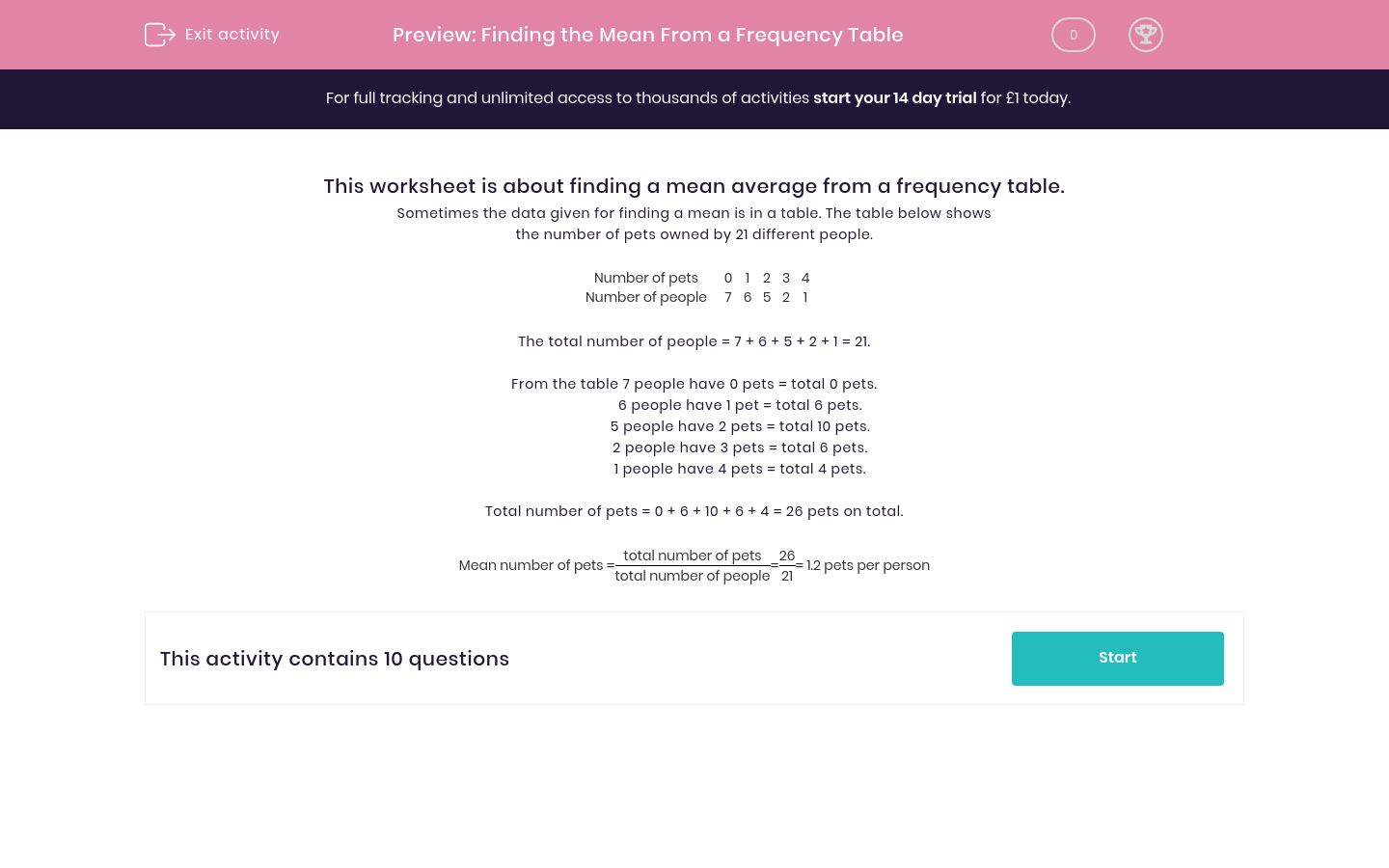# Finding the Mean From a Frequency Table

In this worksheet, students find the mean from a frequency table.Key stage:  KS 2

Curriculum topic:   Maths and Numerical Reasoning

Curriculum subtopic:   Interpreting Data

Difficulty level:#### Worksheet Overview

This worksheet is about finding a mean average from a frequency table.

Sometimes the data given for finding a mean is in a table. The table below shows the number of pets owned by 21 different people.

 Number of pets 0 1 2 3 4 Number of people 7 6 5 2 1

The total number of people = 7 + 6 + 5 + 2 + 1 = 21.

From the table 7 people have 0 pets = total 0 pets.
6 people have 1 pet = total 6 pets.
5 people have 2 pets = total 10 pets.
2 people have 3 pets = total 6 pets.
1 people have 4 pets = total 4 pets.

Total number of pets = 0 + 6 + 10 + 6 + 4 = 26 pets on total.

Mean number of pets =
 total number of pets total number of people
=
 26 21
= 1.2 pets per person

### What is EdPlace?

We're your National Curriculum aligned online education content provider helping each child succeed in English, maths and science from year 1 to GCSE. With an EdPlace account you’ll be able to track and measure progress, helping each child achieve their best. We build confidence and attainment by personalising each child’s learning at a level that suits them.

Get started